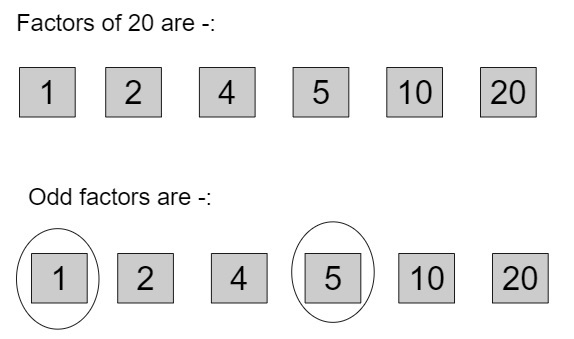# C++ program for Find sum of odd factors of a number

Given with a positive integer and the task is to generate the odd factors of a number and finding out the sum of given odd factors.

## Example

Input-: number = 20
Output-: sum of odd factors is: 6
Input-: number = 18
Output-: sum of odd factors is: 13So, result = 1 + 5 = 6

Approach used in the below program is as follows

• Input the number for calculating the sum of odd factors of that number
• Ignore the digit 0 and 2 because both are even digits and store the digit 1 because it is an odd digit
• Start the loop from 3 till square root of a number
• Traverse till number % i is returning 0 and keep dividing the number with the value of i
• In the loop keep setting the value of temporary variable to temp = temp * i
• Set the total to total + temp
• Return the value of final res variable and print the result

## Algorithm

START
Step 1-> Declare function to calculate sum of odd factors
int sum(int num)
declare int res = 1
Loop While(num % 2 == 0)
set num = num / 2
End
Loop For int i = 3 and i <= sqrt(num) and i++
declare int count = 0 and total = 1
declare int temp = 1
Loop while (num % i == 0)
count++
set num = num / i
set temp *= i
set total += temp
End
set res = res*total
End
IF (num >= 2)
set res *= (1 + num)
End
return res
Step 2-> In main()
Declare int num = 20
call sum(num)
STOP

## Example

#include <bits/stdc++.h>
using namespace std;
//calculate sum of odd factors
int sum(int num) {
int res = 1;
while (num % 2 == 0)
num = num / 2;
for (int i = 3; i <= sqrt(num); i++) {
int count = 0, total = 1 ;
int temp = 1;
while (num % i == 0) {
count++;
num = num / i;
temp *= i;
total += temp;
}
res = res*total;
}
if (num >= 2)
res *= (1 + num);
return res;
}
int main() {
int num = 20;
cout<<"sum of odd factors is : ";
cout <<sum(num);
return 0;
}

## Output

sum of odd factors is : 6